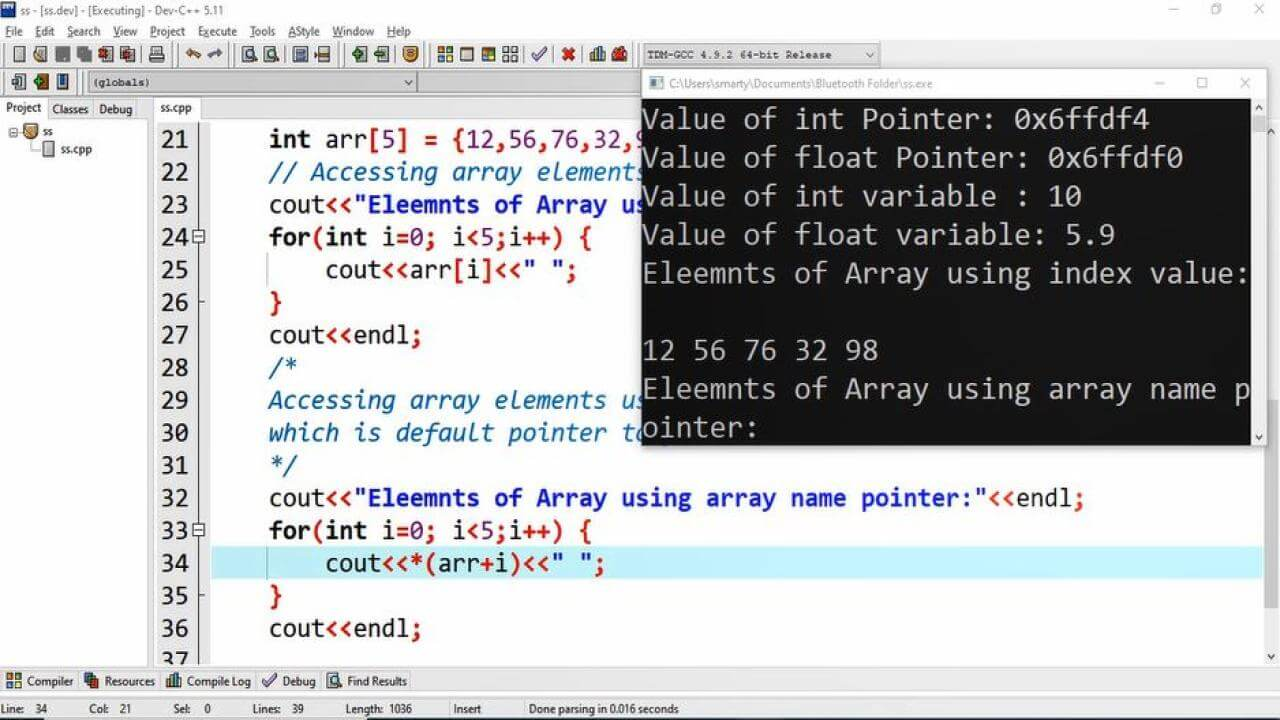# Pointer In C++ Programming source code

## Source Code:

#include <iostream>
using namespace std;

int main(int argc, char** argv) {
// initialization of int and float variables.
int i_var = 10;
float f_var = 5.9;
// initialization of int and float pointers.
int *i_ptr = &i_var;
float *f_ptr = &f_var;

// print values of pointers
cout<<"Value of int Pointer: "<<i_ptr<<endl;
cout<<"Value of float Pointer: "<<f_ptr<<endl;

// print values of variables using pointers.
cout<<"Value of int variable : "<<*i_ptr<<endl;
cout<<"Value of float variable: "<<*f_ptr<<endl;

// initialization of int type array
int arr = {12,56,76,32,98};
// Accessing array elements using array index.
cout<<"Eleemnts of Array using index value:"<<endl;
for(int i=0; i<5;i++) {
cout<<arr[i]<<" ";
}
cout<<endl;
/*
Accessing array elements using array name
which is default pointer to first index in C++.
*/

cout<<"Eleemnts of Array using array name pointer:"<<endl;
for(int i=0; i<5;i++) {
cout<<*(arr+i)<<" ";
}
cout<<endl;

return 0;
}

##Working:

In this program example we have declared two variables of int and float type; Similary have declared and initialized two pointers of int and float type in the next statements. Here is the detail of these variables.

 Sr. Identifie Type Initial Value Size 1 i_var int variable 10 4 2 f_var float variable 5.9 4 3 i_ptr int pointer address of int variable i_var 8 4 f_ptr float pointer address of float variable f_var 8 5 arr int array random 5 values 20

Secondly we declare an array of 5 element of integer data type. Array name is pointer to its first element by default in C++. So that in in for loop we use array name as pointer to access the array elements one after another.